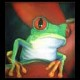# 2D Reflective Water

A Shader to create a reflective Water Effect in 2D. Apply it to a ColorRect Node which is the size of the canvas.

``````// Kitchen Games / 2D Reflective Water Shader

uniform float horizon : hint_range(0.0,1.0); // The height of the horizon
uniform sampler2D noise; // add two noises. Play with these some time to get a decent result
uniform sampler2D noise2;
uniform float wave_frequency : hint_range(0.0, 100.0);
uniform float wave_magnitude : hint_range(0.0, .3);
uniform float tides_magnitude : hint_range(0.0, .3);
uniform float noise_wave : hint_range(0.0, 3.0); // add noisiness to waves
uniform float tides_speed : hint_range(0.0, 20.0);
uniform float wave_speed : hint_range(0.0, 20.0);

uniform float shine_position : hint_range(0.0, 1.0); // configure a sunshine if you want
uniform float shine_itensity : hint_range(0.0, 1.0);
uniform float shine_width : hint_range(0.0, 1.0);
uniform vec4 shine_color : hint_color;

uniform vec4 water_color : hint_color;

void fragment()
{
vec2 noise_uv = vec2(SCREEN_UV.x + TIME * 0.025, SCREEN_UV.y);
float noise_val = texture(noise, noise_uv).r * texture(noise2, SCREEN_UV).r + 0.2;

float wave = horizon;
wave += sin(UV.x * wave_frequency + TIME* wave_speed) * wave_magnitude;
wave += sin(TIME * tides_speed) * tides_magnitude;
wave -= texture(noise2, SCREEN_UV).r * 0.05 * noise_wave;

vec2 offset = vec2(0, (wave + abs(SCREEN_UV.y - wave)) - SCREEN_UV.y);

offset += noise_val* 0.1 - 0.05;
vec2 col_uv = SCREEN_UV;

col_uv.y = col_uv.y + (offset.y * step(SCREEN_UV.y, wave));

vec4 col = texture(SCREEN_TEXTURE, col_uv);
col = mix(col, water_color,  step(SCREEN_UV.y, wave) * 0.5);
col = mix(col, shine_color, step(SCREEN_UV.y, wave) * (step(noise_val + abs(SCREEN_UV.x - shine_position), shine_width)) * shine_itensity);
COLOR = col;
}``````
###### Tags
Water Reflection WaveThe shader code and all code snippets in this post are under CC0 license and can be used freely without the author's permission. Images and videos, and assets depicted in those, do not fall under this license. For more info, see our License terms.

### Water-like waveletInline Feedbacksjun
8 months ago

but……how can i use it? i add it to a Control Node and Nothing happenedCC Cosmos
7 months ago

Add it to a colorRect as the child of a Canvas Layer. (That trick works for basically every Screen Texture/UV based shader like this)

This particular shader changes its perspective drastically based on your camera position. Make sure to tweak it by looking at it in-game to see what it looks like relative to the in-game camera.JUN
7 months ago